Next: Fermi-Dirac statistics Up: Quantum statistics Previous: An illustrative example

## Formulation of the statistical problem

Consider a gas consisting ofidentical non-interacting particles occupying volumeand in thermal equilibrium at temperature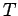. Let us label the possible quantum states of a single particle by(or). Let the energy of a particle in statebe denoted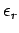. Let the number of particles in statebe written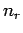. Finally, let us label the possible quantum states of the whole gas by.

The particles are assumed to be non-interacting, so the total energy of the gas in state, where there areparticles in quantum state, etc., is simply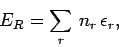(579)

where the sum extends over all possible quantum states. Furthermore, since the total number of particles in the gas is known to be, we must have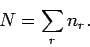(580)

In order to calculate the thermodynamic properties of the gas (i.e., its internal energy or its entropy), it is necessary to calculate its partition function,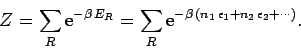(581)

Here, the sum is over all possible statesof the whole gas: i.e., over all the various possible values of the numbers.

Now,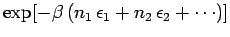is the relative probability of finding the gas in a particular state in which there are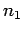particles in state 1,particles in state 2, etc. Thus, the mean number of particles in quantum statecan be written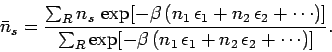(582)

A comparison of Eqs. (581) and (582) yields the result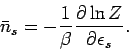(583)

Here,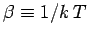.Next: Fermi-Dirac statistics Up: Quantum statistics Previous: An illustrative example
Richard Fitzpatrick 2006-02-02